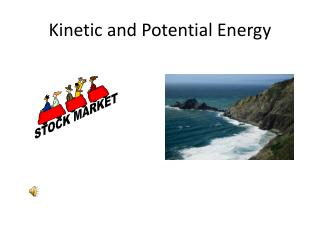DownloadDownload PresentationKinetic and Potential Energy

# Kinetic and Potential Energy

Download Presentation## Kinetic and Potential Energy

- - - - - - - - - - - - - - - - - - - - - - - - - - - E N D - - - - - - - - - - - - - - - - - - - - - - - - - - -
##### Presentation Transcript

1. Kinetic and Potential Energy

2. Kinetic Energy • Kinetic energy is the energy of movement or motion • To calculate kinetic energy you need to know an object’s mass and velocity • Kinetic energy (KE) = ½ (m)*(v2) = ½ mv2 • Energy is expressed in Joules (J) which are kg*m2/s2

3. Example how to calculate kinetic energy A 0.10 kg bird is flying at a constant speed of 8.0m/s. What is the bird’s kinetic energy? Mass (m) = .10kg Speed (v) = 8.0m/s2 KE = ½ (.10kg)* (8.0m/s)2 KE = 3.2 kg*m2/s2 = 3J

4. Kinetic Energy Equations • If you are asked to solve for kinetic energy use • KE = ½ mv2 • If you are asked to solve for mass use • M = 2*(KE)/v2 • If you are asked to solve for velocity use • V = square root 2KE/m

5. Potential Energy • Potential energy is the energy stored as a result of position • Gravitational potential energy depends on an object’s height

6. Formula for calculating potential energy • The formula is PEg = mgh = Fgh • Fg will be in Newtons • Where m = mass, g = gravity and h = height • In the second form • F = force in newtons(N), h = height • Gravitational potential energy is expressed in Joules (J)

7. Example of calculating gravitational potential energy • A diver at the top of a 10.0 m high diving platform has a mass of 50.0kg. What is her potential energy? • PE = mgh • PE = (50.0kg)*(9.8m/s2)*(10.0m) • PE = 4900 kg*m2/s2 = 4900J

8. Potential Energy Equations • If you are asked to solve for potential energy use • PE = mgh or PE = Fgh • If you are asked to solve for mass use • m = PE/gh or m = PE/Fgh • If you are asked to solve for height use • h = PE/mg or use h = PE/Fg

9. Elastic Potential Energy • Elastic potential energy is the energy stored in an object that is stretched or compressed (think elastic) • The energy is stored when the object is either stretched or compressed

10. Chemical Energy • Chemical energy is the energy stored in chemical bonds • The energy is released when the bonds are broken

11. Electric Energy • Electric energy is the energy associated with electric charges • Batteries contain electric energy

12. Nuclear Energy • Nuclear energy is the energy stored in the nucleus of an atom • Energy can be released by nuclear fission which is splitting the atom or by nuclear fusion which is joining nuclei